It is often useful to explore a data set visually to get a sense of what information it contains. It can be helpful to look at a data set from different perspectives.

Hadley Wickham’s R for Data Science includes a number of exercises designed to illustrate the ggplot function’s various arguments and how they can be used to look at a single data set in a variety of ways.

It is helpful to explore data visually by playing with the arguments to see which combinations best illustrate what the data are saying.

The following examples are drawn from this excellent book. You can find the code here!

``library(ggplot2)``

Let’s take a look at the mpg data set included in the package. We can see that we have 11 variables to work with and 234 observations. Some of these variables appear to be qunatitative (such as city mpg) while others are qualitative (drive configuration).

``str(mpg)``
``````## Classes 'tbl_df', 'tbl' and 'data.frame':    234 obs. of  11 variables:
##  \$ manufacturer: chr  "audi" "audi" "audi" "audi" ...
##  \$ model       : chr  "a4" "a4" "a4" "a4" ...
##  \$ displ       : num  1.8 1.8 2 2 2.8 2.8 3.1 1.8 1.8 2 ...
##  \$ year        : int  1999 1999 2008 2008 1999 1999 2008 1999 1999 2008 ...
##  \$ cyl         : int  4 4 4 4 6 6 6 4 4 4 ...
##  \$ trans       : chr  "auto(l5)" "manual(m5)" "manual(m6)" "auto(av)" ...
##  \$ drv         : chr  "f" "f" "f" "f" ...
##  \$ cty         : int  18 21 20 21 16 18 18 18 16 20 ...
##  \$ hwy         : int  29 29 31 30 26 26 27 26 25 28 ...
##  \$ fl          : chr  "p" "p" "p" "p" ...
##  \$ class       : chr  "compact" "compact" "compact" "compact" ...``````

The tabular format really doesn’t help us understand this data set. So let’s begin to slice and dice the data and see what we cab learn. We might suspect there is a relationship between highway mileage and the size of the engine:

``````ggplot(data = mpg) + geom_point(mapping = aes(x = displ, y = hwy)) +
ggtitle("Displacement and Highway Mileage") +
labs(x = "engine displacement (in liters)", y = "highway mileage/gallon")``````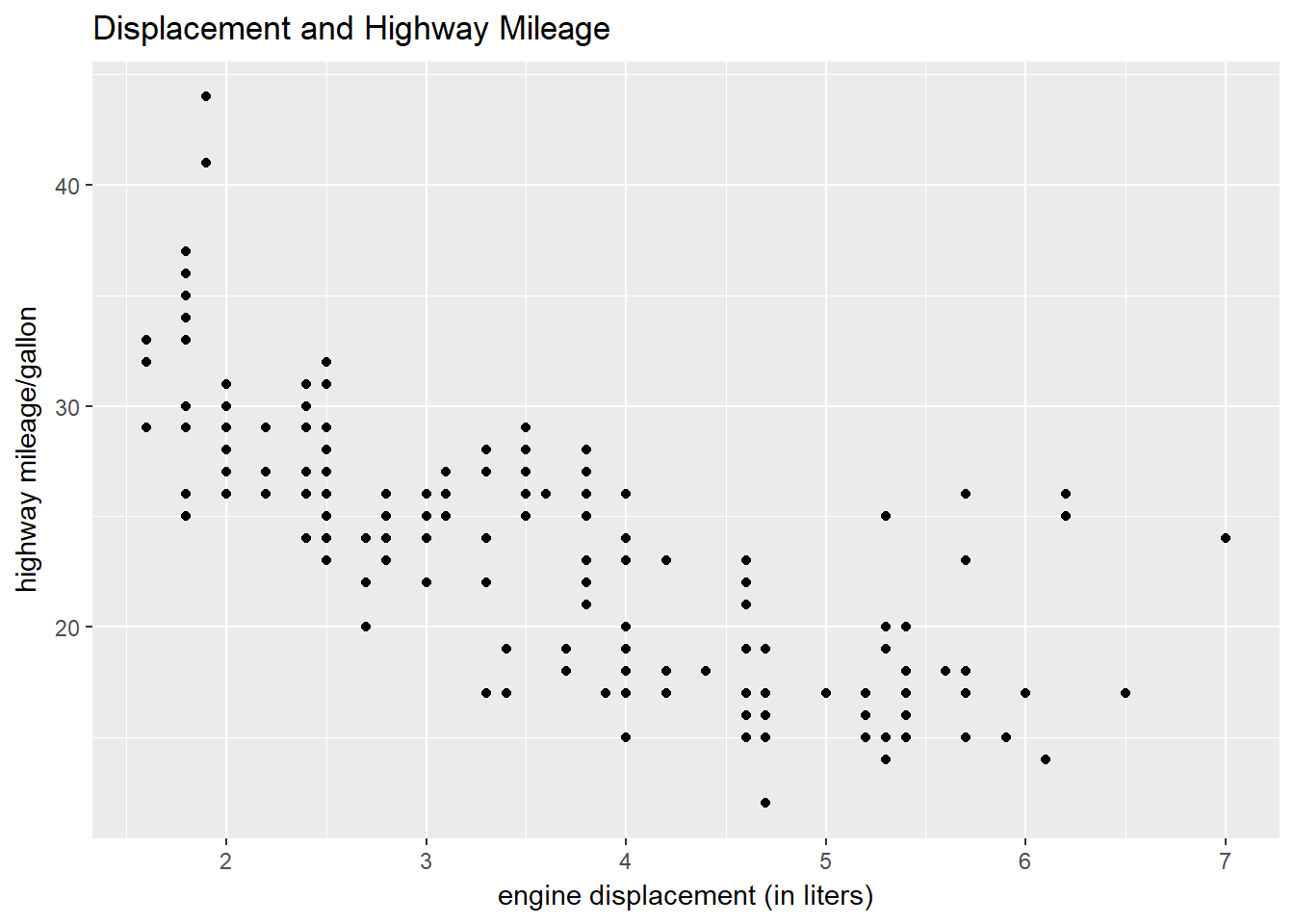There is something odd about the preceding graph. All the data point line up in straight columns. But that’s not how numbers typically behave. It turns out our x and y values are rounded, so the data point line up vertically. Many overlap.

We can work around this by using geom_jitter in place of geom_point. We add the width and height specification in order to control the amount of “jitter,” which is simply a random values added to the data points to avoid overlap. See what happens when you set both to zero.

``````ggplot(data = mpg) + geom_jitter(mapping = aes(x = displ, y = hwy), width = 0.25, height = 0.25) +
ggtitle("Displacement and Highway Mileage") +
labs(x = "engine displacement (in liters)", y = "highway mileage/gallon")``````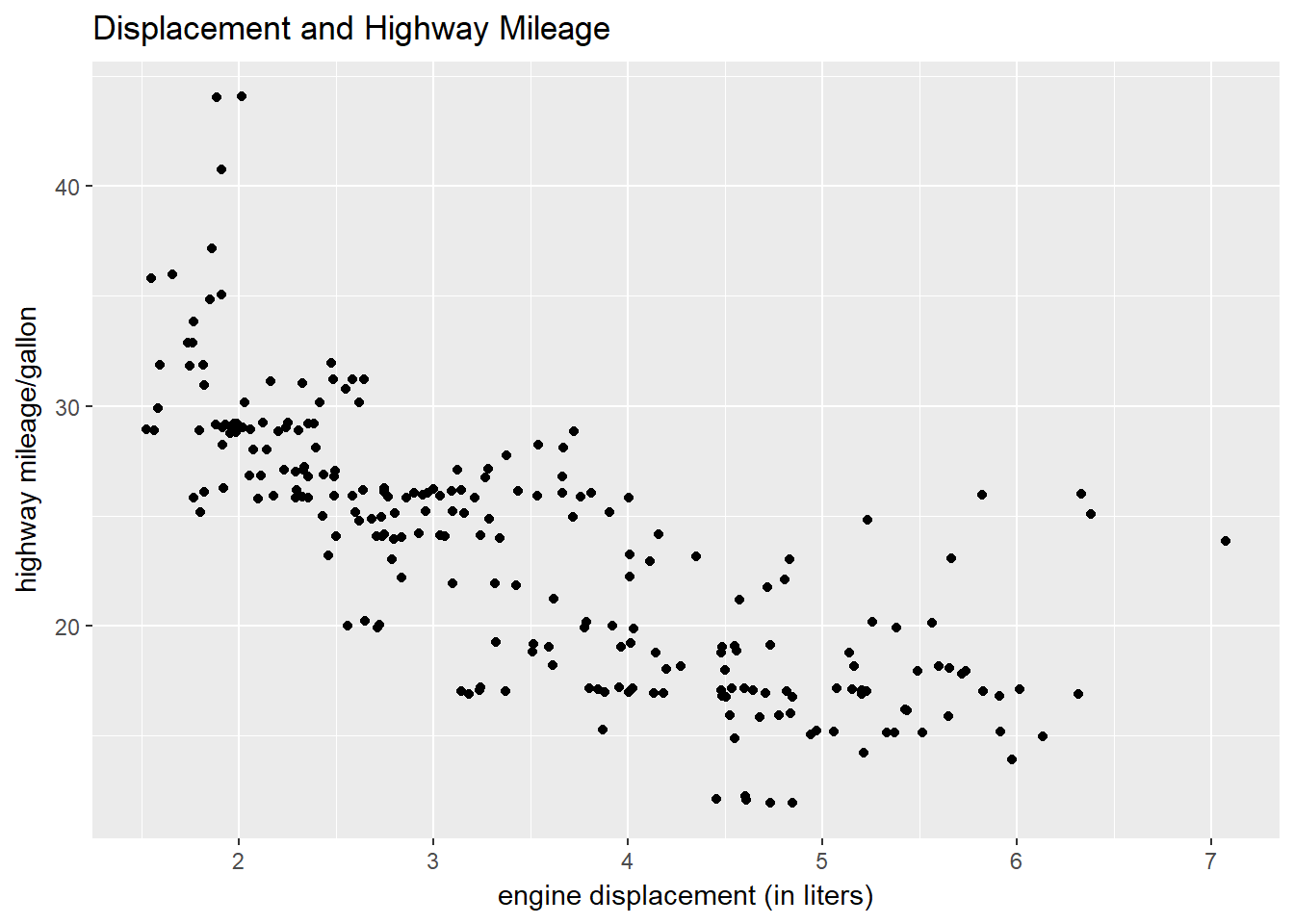We can see the relationship here between two variables. But suppose we want to add a third? Let’s try to identify each data point by the class of vehicle it represents. To do this, we add the alpha argument to the aesthetic we are using. Alpha uses transparency levels to distinguish classes.

``````ggplot(data = mpg) + geom_point(mapping = aes(x = displ, y = hwy, alpha = class)) +
ggtitle("Displacement and Highway Mileage") +
labs(x = "engine displacement (in liters)", y = "highway mileage/gallon")``````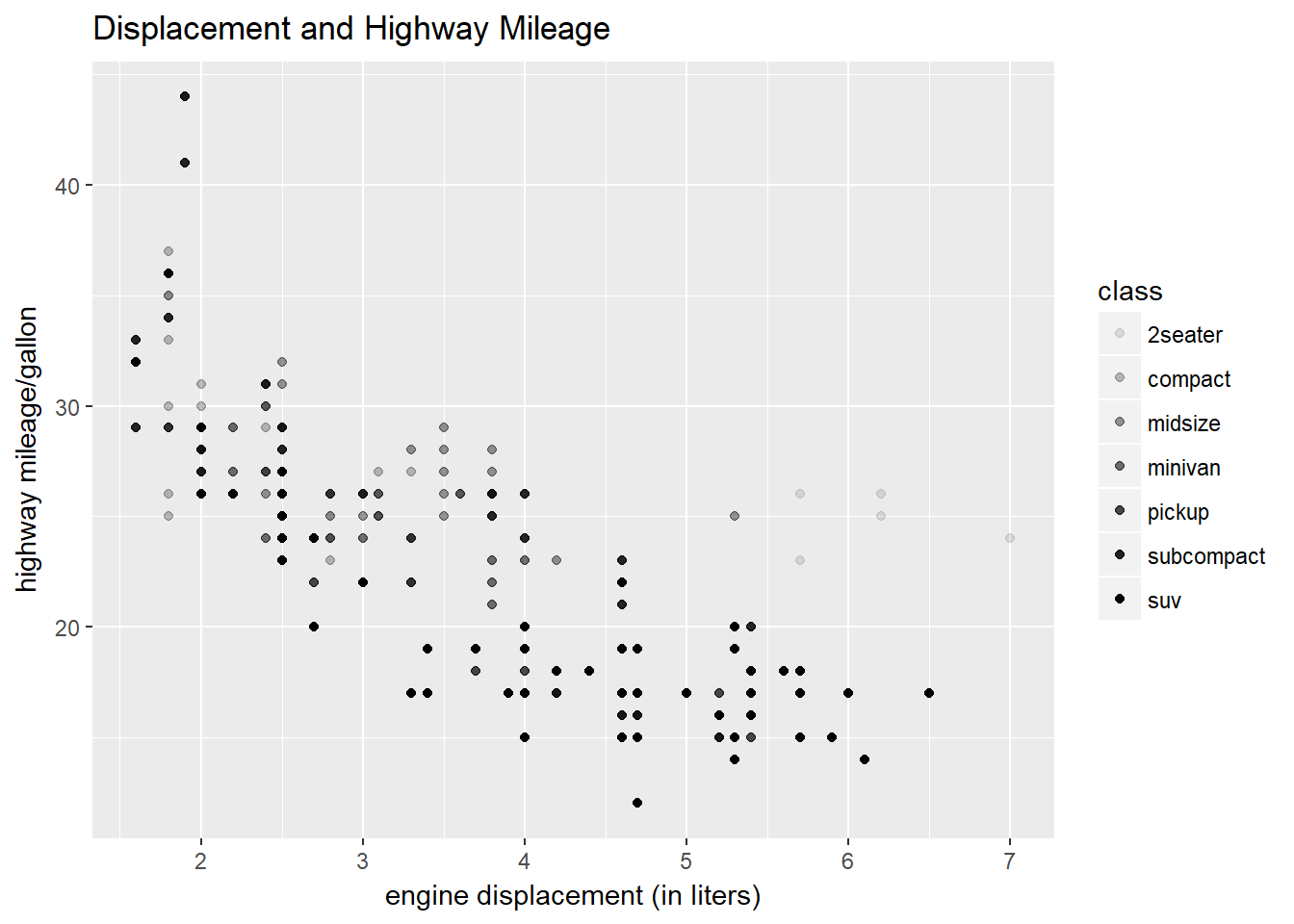That helps a bit, but the grays kind of wash out and don’t make the classes as distinct as we would like. Adding color helps solve this problem.

``````ggplot(data = mpg) + geom_point(mapping = aes(x = displ, y = hwy, color = class)) +
ggtitle("Displacement and Highway Mileage") +
labs(x = "engine displacement (in liters)", y = "highway mileage/gallon")``````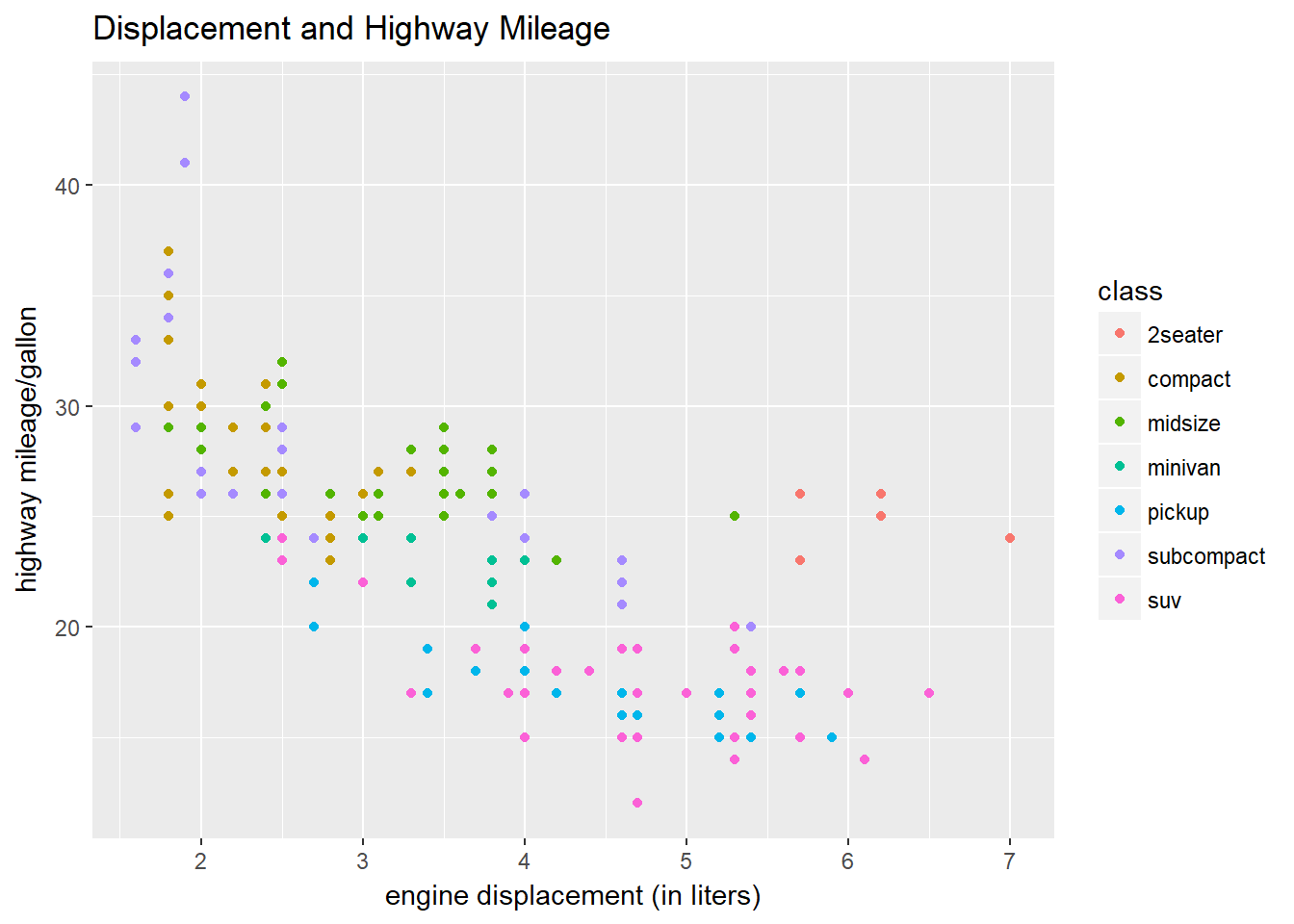It is also possible in ggplot2 to use shapes to distinguish data points and classes. This works best for six classes, so in our example we will throw a warning and lose some data.

``````ggplot(data = mpg) + geom_point(mapping = aes(x = displ, y = hwy, shape = class)) +
ggtitle("Displacement and Highway Mileage") +
labs(x = "engine displacement (in liters)", y = "highway mileage/gallon")``````
``````## Warning: The shape palette can deal with a maximum of 6 discrete values
## because more than 6 becomes difficult to discriminate; you have 7.
## Consider specifying shapes manually if you must have them.``````
``## Warning: Removed 62 rows containing missing values (geom_point).``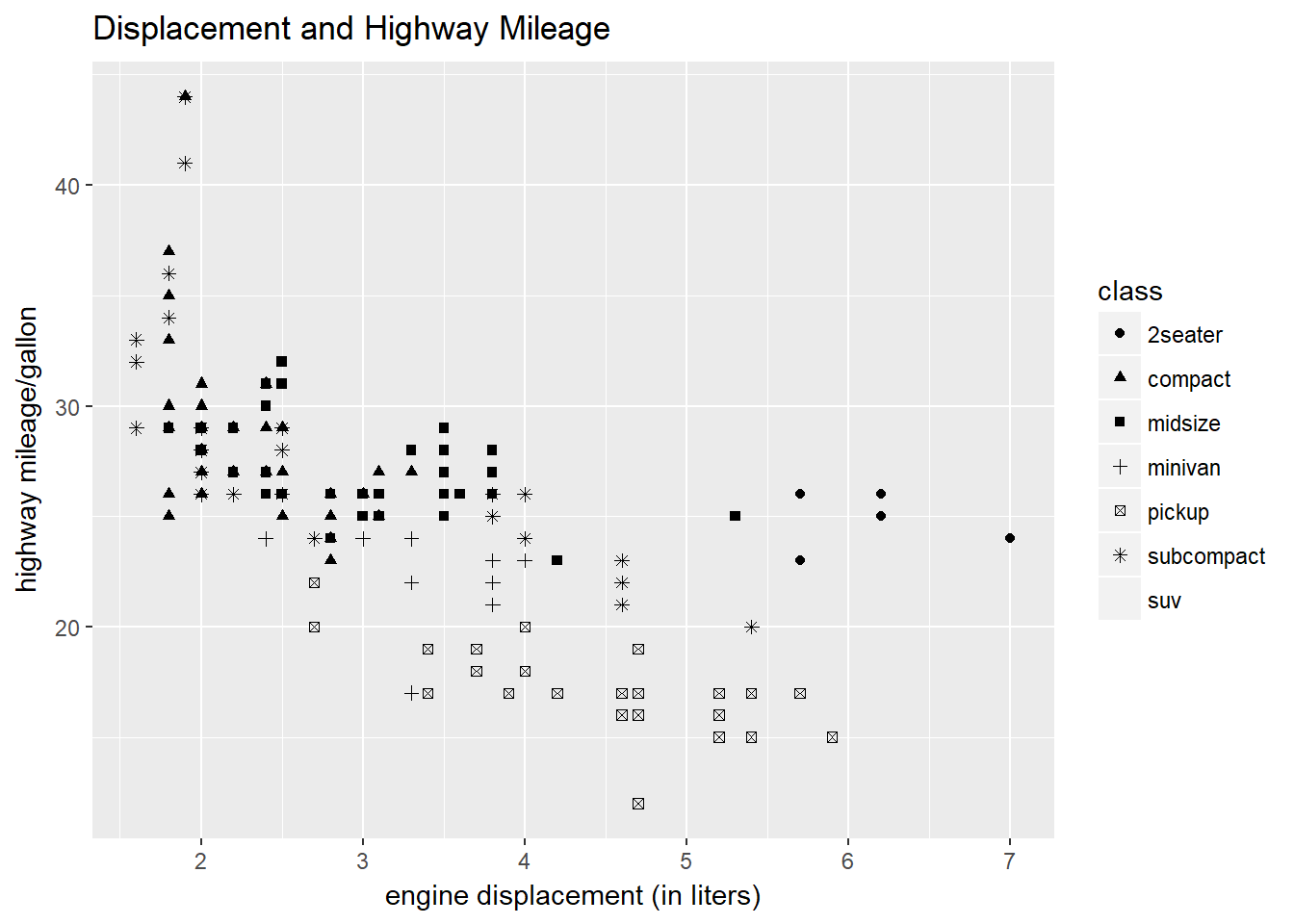Another argument we can use in ggplot2 to introduce a third variable (and a fourth) is facet. We will use two flavors to illustrate this: facet_wrap and facet_grid. As you will see, it’s not necessary to include color to distinguish class, but it does make the graphic more appealing.

``````ggplot(data = mpg) + geom_point(mapping = aes(x = displ, y = hwy, color = class)) +
ggtitle("Displacement and Highway Mileage") +
labs(x = "engine displacement (in liters)", y = "highway mileage/gallon") +
facet_wrap( ~ class, nrow = 4)``````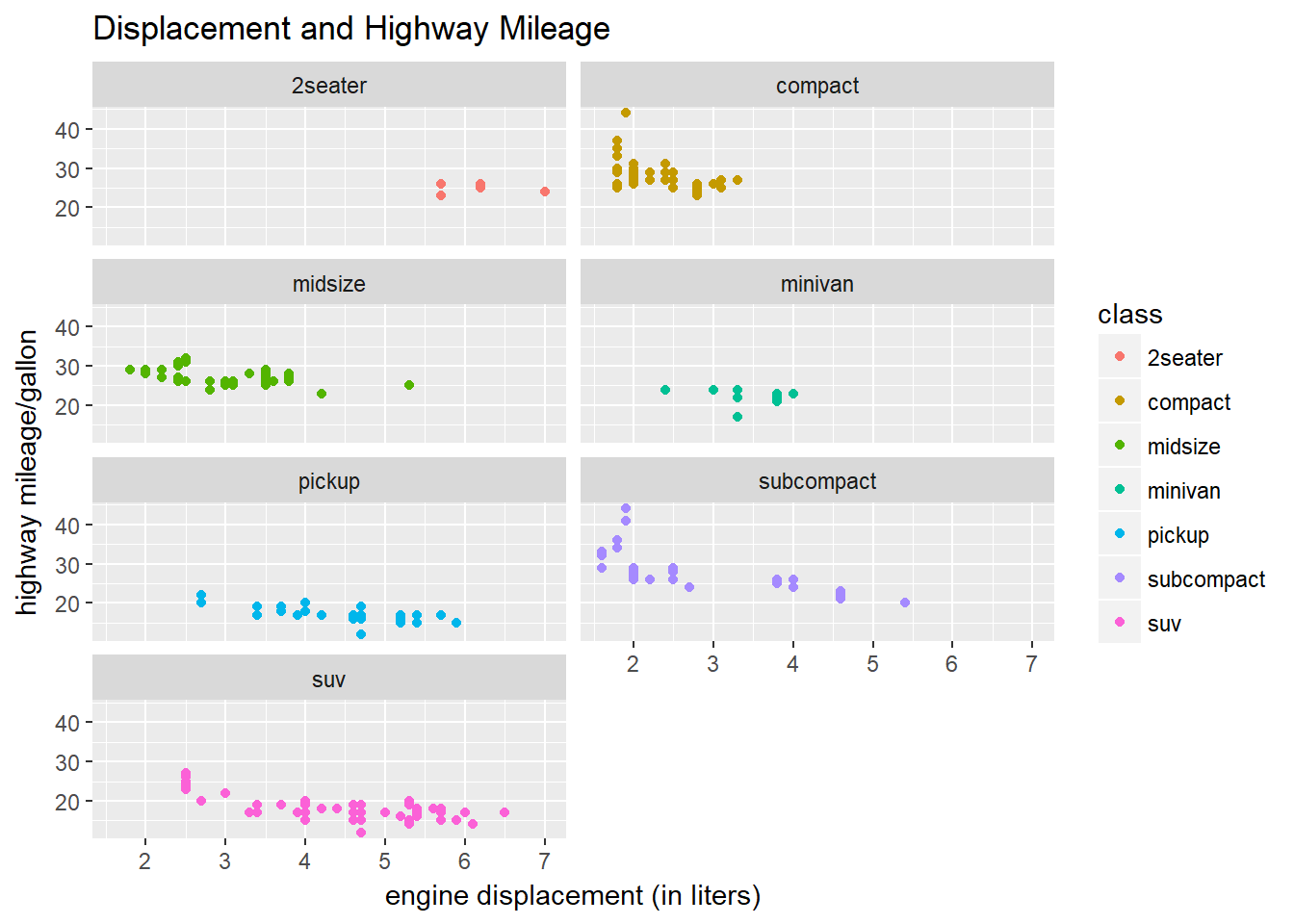Now let’s introduce a fourth variable to our plot. Color becomes more useful if we make the same plot but use another variable (such as drv) instead of class.

``````ggplot(data = mpg) + geom_point(mapping = aes(x = displ, y = hwy, color = class)) +
ggtitle("Displacement and Highway Mileage") +
labs(x = "engine displacement (in liters)", y = "highway mileage/gallon") +
facet_wrap( ~ drv, nrow = 3)``````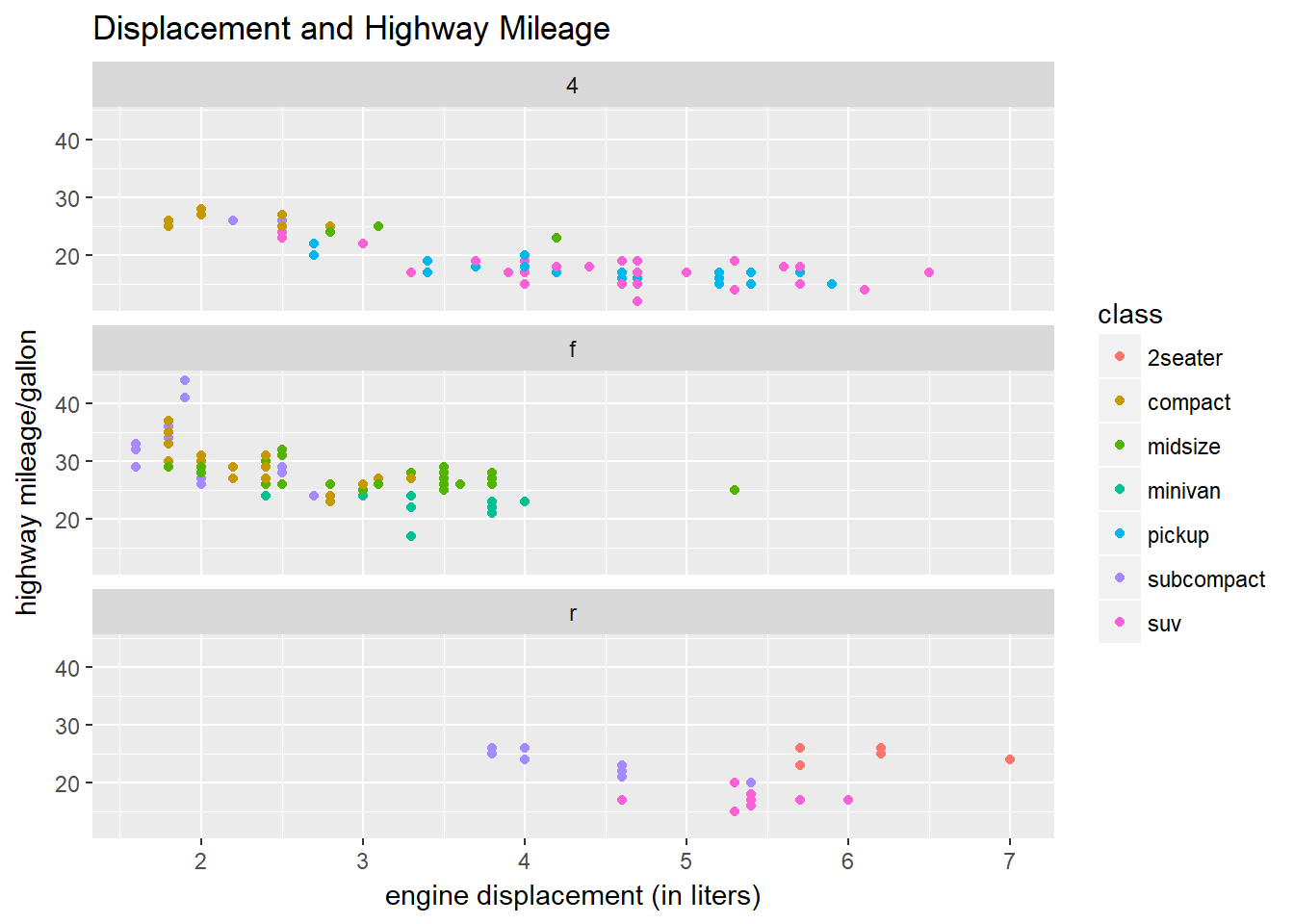We can create the same plot using the facet_grid argument.

``````ggplot(data = mpg) + geom_point(mapping = aes(x = displ, y = hwy, color = class)) +
ggtitle("Displacement and Highway Mileage") +
labs(x = "engine displacement (in liters)", y = "highway mileage/gallon") +
facet_grid(drv ~ .)``````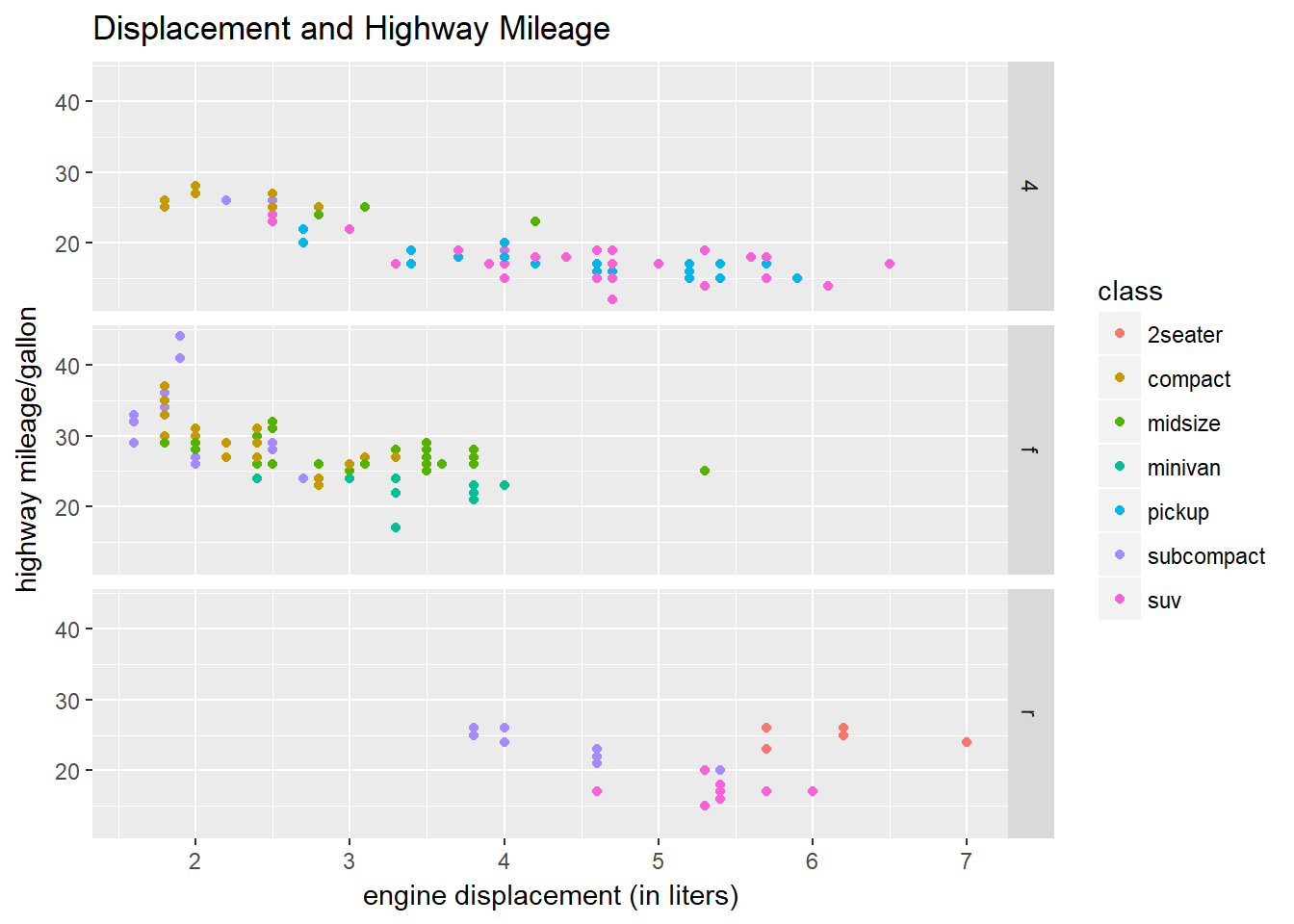Let’s try another fourth variable and orient it vertically We do this by putting the variable cyl in the y-axis position (. ~ cyl).

``````ggplot(data = mpg) + geom_point(mapping = aes(x = displ, y = hwy, color = class)) +
ggtitle("Displacement and Highway Mileage") +
labs(x = "engine displacement (in liters)", y = "highway mileage/gallon") +
facet_grid(. ~ cyl)``````